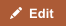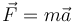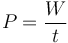The concepts of force and power seem to convey similar meanings and are often confused for each other. But in physics, they are not interchangeable. Force is the fundamental result of an interaction between two objects, while power is an expression of energy consumed over time (work), of which force is an element. Force and power can both be described and measured, but a force is an actual physical phenomenon, and power in itself is not.

## Comparison chart

Force versus Power comparison chartForcePower
Definition A push or a pull resulting from an interaction between objects. Power is the rate at which work is done, or energy is transmitted.
Unit newton watt = joules/second
Symbol F P
Named After Isaac Newton James Watt
Derivations from other quantities F = m a (force = mass multiplied by acceleration) P = w/t (power = work divided by time)
Relation to “Work” Force applied over a distance creates work. Rate at which work is performed.
Everyday Example Gravity, friction, magnetism. Horsepower (1 horsepower = 750 Watts).

## Basic Concept

A force can only occur when objects are interacting. Whenever objects interact, they push or pull one another, whether through direct contact or at-a-distance contact. Examples of direct contact forces include the friction of car tires on a road, or the air resistance on a moving car. At-a-distance interaction occurs through forces like gravity and magnetism. Force is a fundamental expression of physical occurrences, as are time and distance.

Power is defined as the amount of energy consumed per unit of time. Another way to put this is: the rate at which “work” is done. Work occurs when there is force causing movement of an object. For example, a person pressing against a brick wall is consuming energy, but no work is being done and no power created because the wall does not move. But if a person pushes on a table and moves it, then there is work. Power expresses how quickly that work is done. So, force is one element of the power equation, along with other basic elements like distance and time.

## Formulae

Force is calculated as a product of mass and gravitational acceleration, and is usually denoted as$\vec{F} = m \vec{a}$

where F is the force, m is mass and a is the gravitational acceleration.

As a rate of change of work done or the energy of a subsystem, power is calculated as:$P = \frac{W}{t}\,$

where P is power, W is work and t is time.

## Example

Force is inherent in the interaction of any and all objects. When a baseball player hits a ball, the bat exerts force on the ball (and vice versa). Planets orbit the sun because of force. To calculate the force in newtons of a baseball that weights 146 g, you simply multiply the mass (in kilograms, so .146) by acceleration (Earth’s gravity is 9.8 meters per second), which equals 1.43 newtons.

If a person runs and then walks up the same flight of stairs, the same amount of work is done both times, but more power is generated while running, because the same amount of work is being performed in less time.

## Units of Measurement

In scientific applications, force is measured in newtons, and in the English system it’s expressed in pounds. The SI unit of force is the newton (N). One newton is the force required to accelerate a one kilogram mass at a rate of one meter per second squared, or kg·m·s−2. One newton is equal to 100,000 dynes.

The SI unit of power is the watt (W). One watt is equal to one joule per second, where joule is the unit of energy. This is the standard unit of measurement, but power can be expressed by any means of energy over time. Another common expression of power is the horsepower, where 1 horsepower is equal to 746 watts.

## Namesake Scientists

Forces cause acceleration (a change in velocity). The standard unit of force was named after Isaac Newton to celebrate his 2nd law, which states, “the acceleration of an object is directly proportional to the force applied...” 1 newton is the amount of forced required to accelerate a mass of 1 kilogram at a rate of 1 meter per second.

James Watt was a Scottish inventor and engineer. Watt created the measurement of horsepower to help explain the power improvements of his steam engine. On account of his great contribution to the efficiency of steam engines, the scientific community decided to honor him by naming the unit of power after him. The watt was added to the SI as a unit of power in 1960.